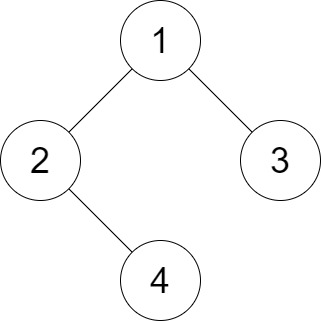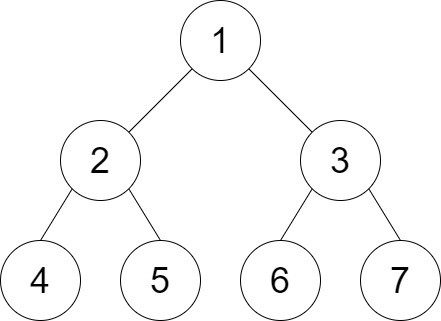Formatted question description: https://leetcode.ca/all/1530.html

# 1530. Number of Good Leaf Nodes Pairs

Medium

## Description

Given the root of a binary tree and an integer distance. A pair of two different leaf nodes of a binary tree is said to be good if the length of the shortest path between them is less than or equal to distance.

Return the number of good leaf node pairs in the tree.

Example 1:Input: root = [1,2,3,null,4], distance = 3

Output: 1

Explanation: The leaf nodes of the tree are 3 and 4 and the length of the shortest path between them is 3. This is the only good pair.

Example 2:Input: root = [1,2,3,4,5,6,7], distance = 3

Output: 2

Explanation: The good pairs are [4,5] and [6,7] with shortest path = 2. The pair [4,6] is not good because the length of ther shortest path between them is 4.

Example 3:

Input: root = [7,1,4,6,null,5,3,null,null,null,null,null,2], distance = 3

Output: 1

Explanation: The only good pair is [2,5].

Example 4:

Input: root = , distance = 1

Output: 0

Example 5:

Input: root = [1,1,1], distance = 2

Output: 1

Constraints:

• The number of nodes in the tree is in the range [1, 2^10].
• Each node’s value is between [1, 100].
• 1 <= distance <= 10

## Solution

Do breadth first search on the binary tree. Use a map to store each leaf node’s path.

After obtaining all leaf nodes, for each pair of leaf nodes, compare their paths and calculate the difference between their paths. If the different is less than or equal to distance, then there is a good leaf nodes pair. Finally, return the number of good leaf nodes pairs.

/**
* Definition for a binary tree node.
* public class TreeNode {
*     int val;
*     TreeNode left;
*     TreeNode right;
*     TreeNode() {}
*     TreeNode(int val) { this.val = val; }
*     TreeNode(int val, TreeNode left, TreeNode right) {
*         this.val = val;
*         this.left = left;
*         this.right = right;
*     }
* }
*/
class Solution {
public int countPairs(TreeNode root, int distance) {
if (root == null || isLeaf(root))
return 0;
Map<TreeNode, String> map = new HashMap<TreeNode, String>();
Queue<TreeNode> nodeQueue = new LinkedList<TreeNode>();
Queue<String> pathQueue = new LinkedList<String>();
nodeQueue.offer(root);
pathQueue.offer("");
while (!nodeQueue.isEmpty()) {
TreeNode node = nodeQueue.poll();
String path = pathQueue.poll();
if (isLeaf(node))
map.put(node, path);
else {
TreeNode left = node.left, right = node.right;
if (left != null) {
nodeQueue.offer(left);
pathQueue.offer(path + "0");
}
if (right != null) {
nodeQueue.offer(right);
pathQueue.offer(path + "1");
}
}
}
int pairs = 0;
List<TreeNode> leafList = new ArrayList<TreeNode>(map.keySet());
int size = leafList.size();
for (int i = 0; i < size - 1; i++) {
TreeNode leaf1 = leafList.get(i);
String path1 = map.get(leaf1);
for (int j = i + 1; j < size; j++) {
TreeNode leaf2 = leafList.get(j);
String path2 = map.get(leaf2);
if (distance(path1, path2) <= distance)
pairs++;
}
}
return pairs;
}

public boolean isLeaf(TreeNode node) {
return node.left == null && node.right == null;
}

public int distance(String path1, String path2) {
int length1 = path1.length(), length2 = path2.length();
int minLength = Math.min(length1, length2);
int differentIndex = -1;
for (int i = 0; i < minLength; i++) {
if (path1.charAt(i) != path2.charAt(i)) {
differentIndex = i;
break;
}
}
if (differentIndex < 0)
return 0;
else
return length1 + length2 - differentIndex * 2;
}
}